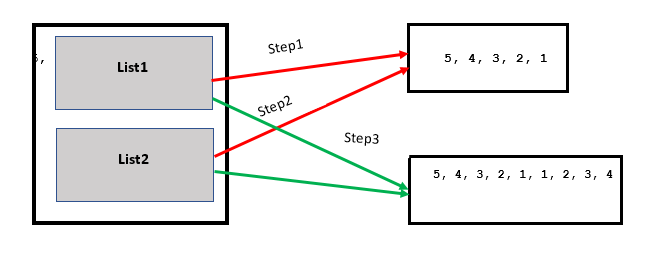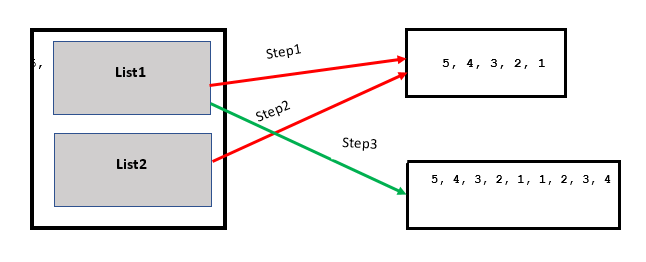# Python | a += b is not always a = a + b

In Python, a += b doesn’t always behave the same way as a = a + b, the same operands may give different results under different conditions. But to understand why they show different behaviors you have to deep dive into the working of variables. before that, we will try to understand the difference between Python Variable and List Reference in Python.

## Difference Between Variable and List Reference

Here, we will see the difference between variable and list reference.

### Creating New Variable

In this example, we have created a new variable and will see about its reference. Here, value 10 gets stored in memory and its reference gets assigned to a.

## Python3

 `a ``=` `10` `print``(``" id of a : "``, ``id``(``10``) ,``" Value : "``, a  )`

Output

```id of a :  11094592  Value :  10
a
or
```

### Modifying The Variable

In this example, we are modifying the variable and then comparing the reference after using + and += operators. Whenever we create or modify int, float, char, or string they create new objects and assign their newly created reference to their respective variables.

## Python3

 `a ``=` `10`  `# Assigning value to variable creates new object` `print``(``" id of a : "``, ``id``(a) ,``" Value : "``, a  ) `   `a ``=` `a ``+` `10` `# Modifying value of variable creates new object` `print``(``" id of a : "``, ``id``(a) ,``" Value : "``, a  )` `  `  `a ``+``=` `10` `# Modifying value of variable creates new object` `print``(``" id of a : "``, ``id``(a) ,``" Value : "``, a  )`

Output

```id of a :  11094592  Value :  10
id of a :  11094912  Value :  20
id of a :  11095232  Value :  30

```

But the same behavior is not seen in the list. In the below example, we can see that the reference remain same when we use + and += operator in the list.

## Python3

 `a ``=` `[``0``, ``1``] ``# stores this array in memory and assign its reference to a` `print``(``"id of a: "``,``id``(a) , ``"Value : "``, a )`   `a ``=` `a ``+` `[``2``, ``3``] ``# this will also behave same store data in memory and assign ref. to variable` `print``(``"id of a: "``,``id``(a) , ``"Value : "``, a )`   `a ``+``=` `[``4``, ``5``] ` `print``(``"id of a: "``,``id``(a) , ``"Value : "``, a )`   `#But now this will now create new ref. instead this will modify the current object so ` `# all the other variable pointing to a will also gets changes`

Output

```id of a:  140266311673864 Value :  [0, 1]
id of a:  140266311673608 Value :  [0, 1, 2, 3]
id of a:  140266311673608 Value :  [0, 1, 2, 3, 4, 5]
```

## Python | a += b is not always a = a + b

In Python, a+=b is not always a=a+b. We will see this aspect with the help of som examples.

Example: In this example, we can see that list2 which is pointing to list1 gets changes without creating a new object. We can see that the content of list1 and list2 are same. It is because we are using a+=b.

## Python3

 `list1 ``=` `[``5``, ``4``, ``3``, ``2``, ``1``]` `list2 ``=` `list1 ` `list1 ``+``=` `[``1``, ``2``, ``3``, ``4``] ``# modifying value in current reference `   `# as on line 4 it modify the value without creating new object ` `# variable list2 which is pointing to list1 gets changes` `print``(list1)` `print``(list2) `

Output

```[5, 4, 3, 2, 1, 1, 2, 3, 4]
[5, 4, 3, 2, 1, 1, 2, 3, 4]
```Example: In this example, we can see that the contents of list1 are same as above but the content of list 2 are different. It is because we are using a=a+b in this example.

## Python3

 `list1 ``=` `[``5``, ``4``, ``3``, ``2``, ``1``]` `list2 ``=` `list1` `list1 ``=` `list1 ``+` `[``1``, ``2``, ``3``, ``4``]`   `# Contents of list1 are same as above ` `# program, but contents of list2 are` `# different.` `print``(list1)` `print``(list2)`

Output

```[5, 4, 3, 2, 1, 1, 2, 3, 4]
[5, 4, 3, 2, 1]

```Whether you're preparing for your first job interview or aiming to upskill in this ever-evolving tech landscape, GeeksforGeeks Courses are your key to success. We provide top-quality content at affordable prices, all geared towards accelerating your growth in a time-bound manner. Join the millions we've already empowered, and we're here to do the same for you. Don't miss out - check it out now!

Previous
Next